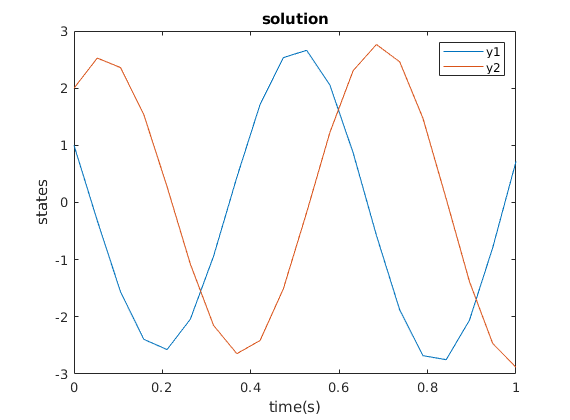# Choose a differents solvers

To start this tutorial, write the following command in the MATLAB console

open T032_SeveralSolvers

In this example, we solve the class ‘ode’ using various numerical methods. By default, it uses the first order forward Euler method for fast calculations. Here, we solve an oscillating system of differential equations:

We may use a linear input to creat an ‘ode’ class:

 \dot Y = AY + BU.

A = [ -5 -10 ; 10 0];
B = [1 ; 1];

dynamics_linear = ode('A',A,'B',B);
dynamics_linear.InitialCondition = [1;2];
[T_default,Y_default] = solve(dynamics_linear);
plot(dynamics_linear)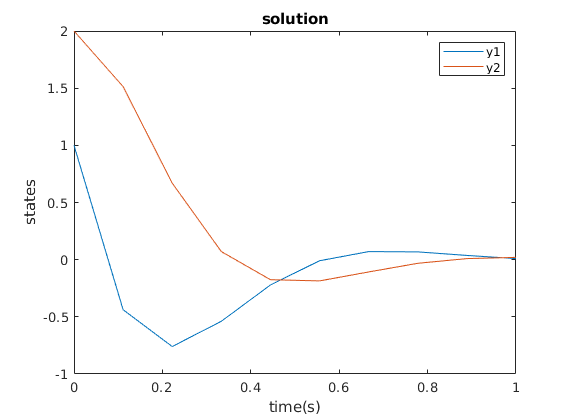‘ode’ class contains the time-descrization information, which we may change manually.

Nt = 20;
dynamics_linear_fine = ode('A',A,'B',B,'Nt',Nt);
dynamics_linear_fine.InitialCondition = [1;2];
[T_Nt20,Y_Nt20] = solve(dynamics_linear_fine);

plot(dynamics_linear_fine)
hold on
plot(T_default,Y_default,'*')
hold off
legend('Y_1','Y_2','Y_1','Y_2')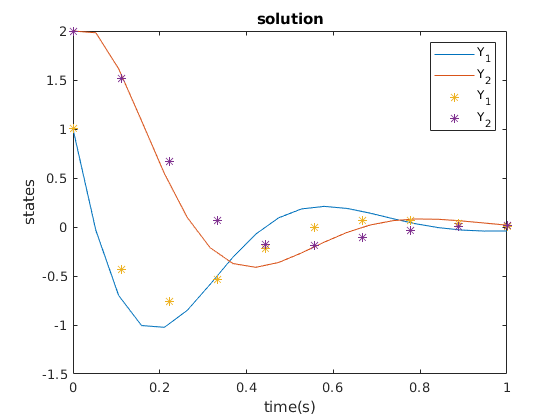‘StateVector.Numeric’ and ‘tspan’ are manual methods to get the results of ‘solve’ function. Note that the values coincide though we gave them different time-steps.

In the (MATLAB built-in) ‘ode45’ function, we may give a (MATLAB built-in) ‘odeset’ class to set the parameters.

odefun = @(t,y) A*y;
tspan = linspace(0,1,Nt);
y0 = [1;2];

options = odeset('RelTol',1e-5,'AbsTol',1e-5);
[t,y] = ode45(odefun,tspan,y0,options);
plot(t,y-Y_Nt20)
xlabel('time(s)')
ylabel('difference of states')
title('Difference of solution with different tolerances')
legend('Y_1','Y_2')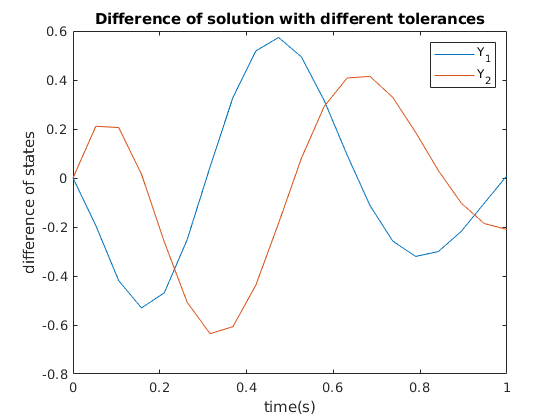We may use ‘ode45’ function to solve the ‘ode’ class and implement the ‘options’ feature of it.

dynamics_linear_fine.SolverParameters = {options};
dynamics_linear_fine.Solver = @ode45;
[T_ode45 Y_ode45] = solve(dynamics_linear_fine);

plot(T_ode45,Y_ode45-y)
xlabel('time(s)')
ylabel('difference of states')
title('Difference of solutions with different classes')
legend('Y_1','Y_2')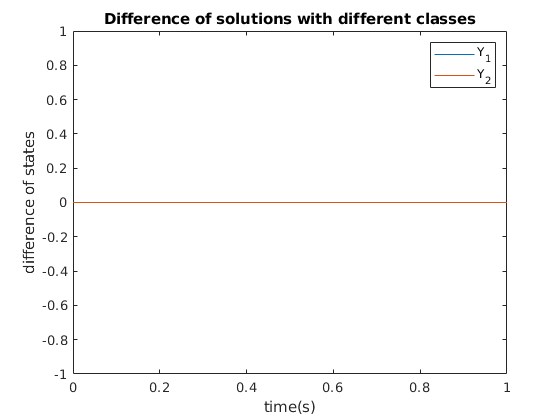Note that the difference of solutions are tiny since they use basically the same numerical methods.

We can use other built-in functions, such as ‘ode23’.

dynamics_linear_23 = ode('A',A,'B',B,'Nt',Nt);
dynamics_linear_23.InitialCondition = [1;2];
dynamics_linear_23.Solver = @ode23;
%%
[T_ode23,Y_ode23] = solve(dynamics_linear_23);

hold on
plot(T_ode23,Y_ode23-Y_ode45)
xlabel('time(s)')
ylabel('difference of states')
title('Difference of solutions with different solvers')
legend('Y_1','Y_2')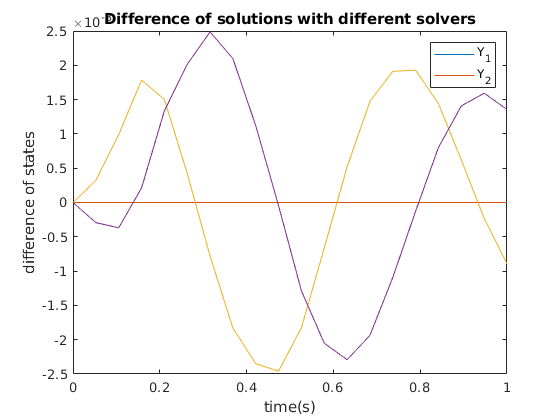In the same way, we may implement a customized function. Here, we may define the first-order Euler method and use it for solving ‘ode’ classes.

dynamics_linear_Euler = ode('A',A,'B',B,'Nt',Nt);
dynamics_linear_Euler.InitialCondition = [1;2];
dynamics_linear_Euler.Solver = @Euler_test;
solve(dynamics_linear_Euler)
plot(dynamics_linear_Euler)

function [tline,yline] = Euler_test(iode)
tline = iode.tspan;
yline = zeros(length(tline),length(iode.StateVector.Symbolic));
yline(1,:) = iode.InitialCondition;
u0    = iode.Control.Numeric;
odefun = iode.DynamicEquation.Num;
for i=1:length(tline)-1
vector = odefun(tline(i),yline(i,:)',u0(i,:)')';
yline(i+1,:) = yline(i,:) + vector*(tline(i+1)-tline(i));
end
end


ans =

Columns 1 through 7

0    0.0526    0.1053    0.1579    0.2105    0.2632    0.3158

Columns 8 through 14

0.3684    0.4211    0.4737    0.5263    0.5789    0.6316    0.6842

Columns 15 through 20

0.7368    0.7895    0.8421    0.8947    0.9474    1.0000